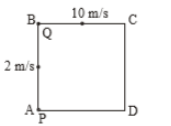Six particles situated at the corners of a regular hexagon of side a move at constant speed v. Each particle maintains a direction towards the particle at the next. The time which the particle will take to meet each other is

1.

2.

3.

4.

Concept Questions :-

Relative motion in 1-D
High Yielding Test Series + Question Bank - NEET 2020

Difficulty Level:

Two men P & Q are standing at corners A & B of square ABCD of side 8 m. They start moving along the track with constant speed 2 m/s and 10 m/s respectively. The time when they will meet for the first time, is equal to:(1) 2 sec

(2) 3 sec

(3) 1 sec

(4) 6 sec

Concept Questions :-

Relative motion in 1-D
High Yielding Test Series + Question Bank - NEET 2020

Difficulty Level:

Two cars A and B are travelling in the same direction with velocities v1 and v2 $\left({v}_{1}>{v}_{2}\right)$. When the car A is at a distance d behind car B, the driver of the car A applied the brake producing a uniform retardation a. There will be no collision when

1. $d<\frac{{\left({v}_{1}-{v}_{2}\right)}^{2}}{2a}$

2. $d<\frac{{v}_{1}^{2}-{v}_{2}^{2}}{2a}$

3. $d>\frac{{\left({v}_{1}-{v}_{2}\right)}^{2}}{2a}$

4. $d>\frac{{v}_{1}^{2}-{v}_{2}^{2}}{2a}$

Concept Questions :-

Relative motion in 1-D
High Yielding Test Series + Question Bank - NEET 2020

Difficulty Level:

An elevator car, whose floor to ceiling distance is equal to 2.7 m, starts ascending with constant acceleration of 1.2 ms–2. 2 sec after the start, a bolt begins falling from the ceiling of the car. The free fall time of the bolt is

(1) $\sqrt{0.54}\text{\hspace{0.17em}}s$

(2) $\sqrt{6}\text{\hspace{0.17em}}s$

(3) 0.7 s

(4) 1 s

Concept Questions :-

Relative motion in 1-D
High Yielding Test Series + Question Bank - NEET 2020

Difficulty Level:

A student is standing at a distance of 50 metres from the bus. As soon as the bus begins its motion with an acceleration of 1ms–2, the student starts running towards the bus with a uniform velocity u. Assuming the motion to be along a straight road, the minimum value of u, so that the student is able to catch the bus is

1. 5 ms–1

2. 8 ms–1

3. 10 ms–1

4. 12 ms–1

Concept Questions :-

Relative motion in 1-D
High Yielding Test Series + Question Bank - NEET 2020

Difficulty Level:

A man is 45 m behind the bus when the bus starts accelerating from rest with acceleration of 2.5 m/s2. With what minimum velocity should the man start running to catch the bus?

1. 12 m/s

2. 14 m/s

3. 15 m/s

4. 16 m/s

Concept Questions :-

Relative motion in 1-D
High Yielding Test Series + Question Bank - NEET 2020

Difficulty Level:

Two trains, each 50 m long are travelling in opposite direction with velocity 10 m/s and 15 m/s. The time of crossing is

(1) 10 s

(2) 4 s

(3) $2\sqrt{3}s$

(4) $4\sqrt{3}s$

Concept Questions :-

Relative motion in 1-D
High Yielding Test Series + Question Bank - NEET 2020

Difficulty Level:

A 120 m long train is moving in a direction with speed 20 m/s. A train B moving with 30 m/s in the opposite direction and 130 m long crosses the first train in a time

(1) 4 s

(2) 36 s

(3) 38 s

(4) 5 s

Concept Questions :-

Relative motion in 1-D
High Yielding Test Series + Question Bank - NEET 2020

Difficulty Level:

A 210 meter long train is moving due North at a speed  of 25m/s. A small bird is flying due South a little above the train with speed 5m/s. The time taken by the bird to cross the train is

(1) 6 s

(2) 7 s

(3) 9 s

(4) 10 s

Concept Questions :-

Relative motion in 1-D
High Yielding Test Series + Question Bank - NEET 2020

Difficulty Level:

A police jeep is chasing with, velocity of 45 km/h a thief in another jeep moving with velocity 153 km/h. Police fires a bullet with muzzle velocity of 180 m/s. The velocity it will strike the car of the thief is

(1) 150 m/s

(2) 27 m/s

(3) 450 m/s

(4) 250 m/s

Concept Questions :-

Relative motion in 1-D